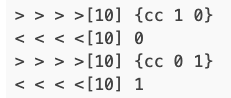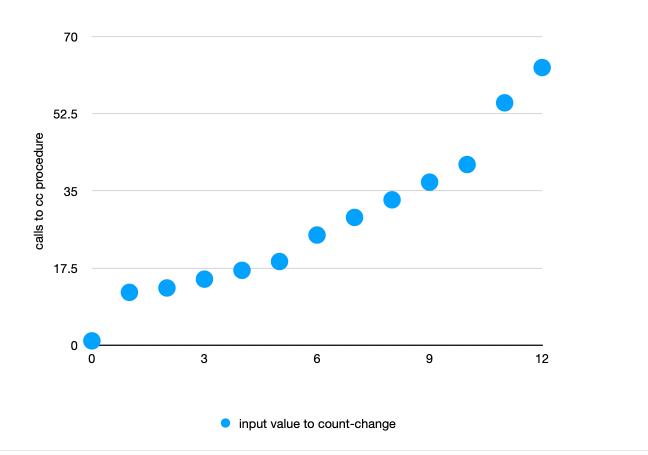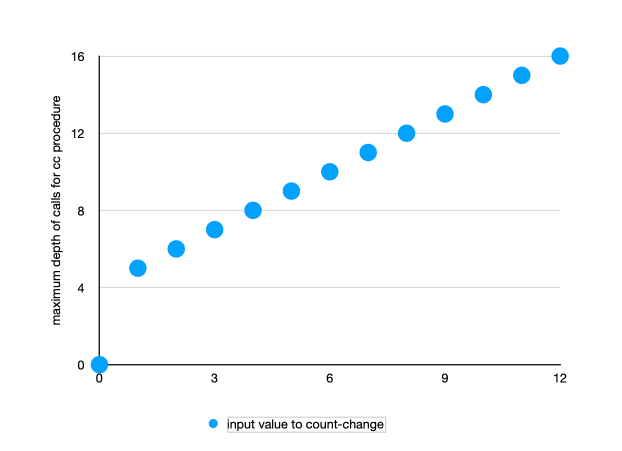# Justin Does SICP

ah now i’m looking again and wondering if your procedure is meant to start counting rows and columns at 1 instead of 0, and so when i gave it unexpected input i caused it to loop

Yes, it was meant to start at 1.

new post

From Section 1.2.3:

I think I get the general idea re: what orders of growth is about but in this more mathematical statement of it, I don’t follow the stuff about the constants

Exercise 1.14: Draw the tree illustrating the process generated by the count-change procedure of 1.2.2 in making change for 11 cents. What are the orders of growth of the space and number of steps used by this process as the amount to be changed increases?

I made trees for (count-change 11) and (count-change 12) (to compare).

I’m planning on looking at these trees again to check for errors (I was having some issues in making them and only came up with an idea to help me error-correct my tree-making when I was mostly done making them).

Anyways, my general impression is that an increase in the amount of change to be made caused some increase in number of computational steps and in “depth” but it didn’t seem explosive like with Fibonacci. So maybe it’s linear? Gonna think about how to analyze this more rigorously.

Exercise 1-14 count-change 11.pdf (77.2 KB)
Exercise 1-14 count-change 12.pdf (60.0 KB)

I think what’s meant here is more like “The sine of an angle (specified in radians) can be computed by making use of the trigonometric identity \sin{x} = 3 \sin{\frac{x}{3}} - 4 \sin^3{\frac{x}{3}} to reduce the size of the argument and also by making use of the approximation \sin{x} ≈ x if x is sufficiently small.” As written it’s confusing.

I had the idea of analyzing the results of trace cc for different values of count-change in order to get a better handle on the procedure’s use of computational resources.

For count-change 6 i got the following:

>(count-change 6)
>{cc 6 5}
> {cc 6 4}
> >{cc 6 3}
> > {cc 6 2}
> > >{cc 6 1}
> > > {cc 6 0}
< < < 0
> > > {cc 5 1}
> > > >{cc 5 0}
< < < <0
> > > >{cc 4 1}
> > > > {cc 4 0}
< < < < 0
> > > > {cc 3 1}
> > > > >{cc 3 0}
< < < < <0
> > > > >{cc 2 1}
> > > > > {cc 2 0}
< < < < < 0
> > > > > {cc 1 1}
> > > > {cc 1 0}
< < < < 0
> > > > {cc 0 1}
< < < < 1
< < < < < 1
< < < < <1
< < < < 1
< < < <1
< < < 1
< < <1
> > >{cc 1 2}
> > > {cc 1 1}
> > > >{cc 1 0}
< < < <0
> > > >{cc 0 1}
< < < <1
< < < 1
> > > {cc -4 2}
< < < 0
< < <1
< < 2
> > {cc -4 3}
< < 0
< <2
> >{cc -19 4}
< <0
< 2
> {cc -44 5}
< 0
<2
2


I’m unsure what the “10” in brackets here signifies and it seems relevant:The Racket page on debugging has some usual info:

18.5 Debugging

When traced procedures invoke each other, nested invocations are shown by printing a nesting prefix. If the nesting depth grows to ten and beyond, a number is printed to show the actual nesting depth.

So how I think it works is like this. The first > in the output returned by a trace indicates 0 depth. So if it’s the initial invocation of the procedure you’re invoking, or something evaluated within the body Then depth is indicated by an alternation between spaces and additional >'s.

So:
>{cc 7 5}
is 0 depth,
> > {cc 7 2}
is 3 depth (space-bracket-space, excluding first bracket), and
> > > > > {cc 2 1}
is 9 depth (5 spaces and 4 brackets excluding first bracket).
Then past that the number in the square brackets indicates the depth and you basically totally ignore the other stuff (the angle brackets and spaces), which don’t actually indicate anything meaningful when there is a number in square brackets.

I had a vague idea about what the trace output indicated before but in order to try to do the sort of analysis I wanted to do here I needed to make it more precise.

This table and chart reflect the number of computational steps (reflected in calls to cc) that result when invoking count-change with a given input

input value to count-change calls to cc
0 1
1 12
2 13
3 15
4 17
5 19
6 25
7 29
8 33
9 37
10 41
11 55
12 63

looks pretty linear to me though with occasionally jumps. I’m not sure if the jumps matter for Order of Growth analysisand the increase in max depth (which, per SICP, is an indicator of the space/memory required when analyzing a tree-recursive process like count-change) is so linear it’s a straight line

input value to count-change
maximum depth of calls for cc procedure
0 0
1 5
2 6
3 7
4 8
5 9
6 10
7 11
8 12
9 13
10 14
11 15
12 16btw i was able to paste the table straight from Numbers and it worked

there’s a pattern in the increases of calls to for higher inputs. Like it starts going up by 1, then by 2, then by 4, and further increases after that.

possibly the pattern of increase would be more clear if i looked at all the computational steps instead of just focusing on cc. i wanted to try that first cuz i wanted to try something simpler. the increase in space seems linear at least

looked at some more data. count-change's number of steps definitely seems to increase massively as the input number increases, though I’m still not confident how to characterize that increase

input value to count-change
calls to cc calls to first-denomination calls to cc + first-denomination
0 1 0 1
1 12 5 17
2 13 6 19
3 15 7 22
4 17 8 25
5 19 9 28
6 25 12 37
7 29 14 43
8 33 16 49
9 37 18 55
10 41 20 61
11 55 23 78
12 63 31 94
20 151 75 226
30 391 195 586
100 15499 7749 23248

Still not feeling confident/clear re: exercise 1.14. Gonna try reading this and maybe some other stuff next How to compute Time Complexity or Order of Growth of any program | Rookie's Lab

Wound up looking at some answers for 1.14.
Been looking at this one and trying to figure it out:

In particular I’ve been trying to figure out the section with the equations that starts with:

T(n,2);=\frac n5+1+;\sum_{i=0}^{n/5}T(n-5i,1)

I looked up Sigma notation and I think I get the idea but I’m still having trouble following all the steps in that solution.

So far my impression is that SICP seems kinda like a math textbook that uses computer science examples rather than the other way around. I know that’s not literally true but that’s how it seems from the perspective of my current skills. (Maybe it’s kinda like, if you don’t know English well then any random English book can feel like a test of vocab/grammar/comprehension)

I’m finding that even reading other people’s solutions to problems seems to have mathematical prerequisites I don’t meet and I’m getting stuck cuz of that. It’s one thing to not be able to come up with a solution on my own but if I’m struggling to understand answers then that seems like a big problem.

It’s possible that I should work on improving my math skills. Idk.

you could try skipping the most mathy problems

1 Like

New post

Hint/help request:

Here is some of Section 1.2.4 as context:

Exercise 1.16: Design a procedure that evolves an iterative exponentiation process that uses successive squaring and uses a logarithmic number of steps, as does fast-expt. (Hint: Using the observation that (b^{n/2})^2=(b^2)^{n/2}, keep, along with the exponent n and the base b, an additional state variable a, and define the state transformation in such a way that the product ab^n is unchanged from state to state. At the beginning of the process a is taken to be 1, and the answer is given by the value of a at the end of the process. In general, the technique of defining an invariant quantity that remains unchanged from state to state is a powerful way to think about the design of iterative algorithms.)

I was a bit confused as to what they were asking for here at first. I found something online which said “The goal is to use the algorithm of fast-exp […] but avoid using the recursive call as an argument to something else.”

Here is a partially pseudocode solution (cuz there is a step missing)

(define (new-expt b n)
(new-expt-iter b n a))

(define (new-expt-iter b n a)
(cond ((= n 0)
a)
((even? n)
(new-expt-iter b (/ n 2)(MAGIC GOES HERE?)))
(else
(new-expt-iter b (- n 1)(* b a)))))


Assuming I am vaguely on the right track, I think what I am missing is how to “define the state transformation in such a way that the product ab^n is unchanged from state to state.”

I wound up starting to read someone else’s answer and now see the problem.

I got stuck on wanting to try to solve the problem without changing the base value b. I think my issue was that I read this part of the hint…

Using the observation that (b^{n/2})^2=(b^2)^{n/2}, keep, along with the exponent n and the base b, an additional state variable a, and define the state transformation in such a way that the product ab^n is unchanged from state to state.

…and for some reason I decided that the base needed to remain constant. I didn’t apply this idea to any of the other values, though.


(define (new-expt b n)
(new-expt-iter b n 1))

(define (new-expt-iter b n a)
(cond ((= n 0)
a)
((even? n)
(new-expt-iter (* b b)(/ n 2) a))
(else
(new-expt-iter b (- n 1)(* b a)))))


I managed to avoid spending lots of time ineffectively spinning my wheels on this. I came back to it a couple of times over some period of time and tried thinking about it some before finally deciding to look at the answer. I’ve also been working on some stuff I think is related (like trying to make sure I’ve actually mastered the Simply Scheme material and working on my basic math skills)